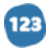# The Traversal Of The Infinite

analytical Essay
1918 words
1918 wordsFiniteness has to do with the existence of boundaries. Intuitively, we feel that where there is a separation, a border, a threshold – there is bound to be at least one thing finite out of a minimum of two. This, of course, is not true. Two infinite things can share a boundary. Infinity does not imply symmetry, let alone isotropy. An entity can be infinite to its “left” – and bounded on its right. Moreover, finiteness can exist where no boundaries can. Take a sphere: it is finite, yet we can continue to draw a line on its surface infinitely. The “boundary”, in this case, is conceptual and arbitrary: if a line drawn on the surface of a sphere were to reach its starting point – then it is finite. Its starting point is the boundary, arbitrarily determined to be so by us. This arbitrariness is bound to appear whenever the finiteness of something is determined by us, rather than “objectively, by nature”. A finite series of numbers is a fine example. WE limit the series, we make it finite by imposing boundaries on it and by instituting “rules of membership”: “A series of all the real numbers up to and including 1000” . Such a series has no continuation (after the number 1000). But, then, the very concept of continuation is arbitrary. Any point can qualify as an end (or as a beginning). Are the statements: “There is an end”, “There is no continuation” and “There is a beginning” – equivalent? Is there a beginning where there is an end ? And is there no continuation wherever there is an end? It all depends on the laws that we set. Change the law and an end-point becomes a starting point. Change it once more and a continuation is available. Legal age limits display such flexible properties. Finiteness is also implied in a series of relationships in the physical world : containment, reduction, stoppage. But, these, of course, are, again, wrong intuitions. They are at least as wrong as the intuitive connection between boundaries and finiteness. If something is halted (spatially or temporally) – it is not necessarily finite. An obstacle is the physical equivalent of a conceptual boundary. An infinite expansion can be checked and yet remain infinite (by expanding in other directions, for instance). If it is reduced – it is smaller than before, but not necessarily finite. If it is contained – it must be smaller than the container but, again, not necessarily fi... ... middle of paper ... ...tes the most serious problem of dealing with the infinite: that our language, our daily experience (=traversal) – all, to our minds, are “finite”. We are told that we had a beginning (which depends on the definition of “we”. The atoms comprising us are much older, of course). We are assured that we will have an end (an assurance not substantiated by any evidence). We have starting and ending points (arbitrarily determined by us). We count, then we stop (our decision, imposed on an infinite world). We put one thing inside another (and the container is contained by the atmosphere, which is contained by Earth which is contained by the Galaxy and so on, ad infinitum). In all these cases, we arbitrarily define both the parameters of the system and the rules of inclusion or exclusion. Yet, we fail to see that WE are the source of the finiteness around us. The evolutionary pressures to survive produced in us this blessed blindness. No decision can be based on an infinite amount of data. No commerce can take place where numbers are always infinite. We had to limit our view and our world drastically, only so that we will be able to expand it later, gradually and with limited, finite, risk.

#### In this essay, the author

• Explains that finiteness has to do with the existence of boundaries. infinity does not imply symmetry, let alone isotropy.
• Explains that an arbitrariness is bound to appear whenever the finiteness of something is determined by us, rather than "objectively, by nature."
• Explains that finiteness is implied in a series of relationships in the physical world — containment, reduction, stoppage. these are, again, wrong intuitions.

•